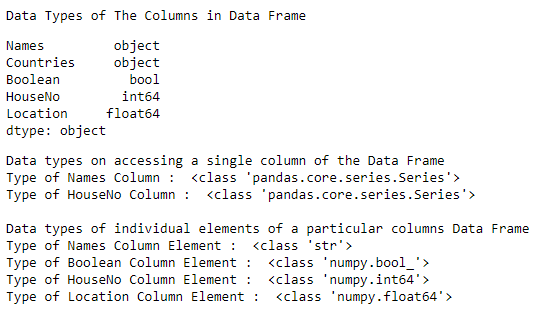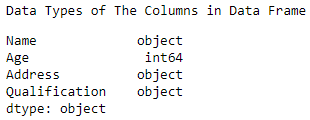Related Articles
Get the datatypes of columns of a Pandas DataFrame
• Last Updated : 17 Aug, 2020

Let us see how to get the datatypes of columns in a Pandas DataFrame. TO get the datatypes, we will be using the `dtype()` and the `type()` function.

Example 1 :

 `# impporting the module``import` `pandas as pd``  ` `# creating a DataFrame    ``dictionary ``=` `{``'Names'``:[``'Simon'``, ``'Josh'``, ``'Amen'``, ``'Habby'``, ``                       ``'Jonathan'``, ``'Nick'``, ``'Jake'``], ``              ``'Countries'``:[``'AUSTRIA'``, ``'BELGIUM'``, ``'BRAZIL'``, ``                           ``'JAPAN'``, ``'FRANCE'``, ``'INDIA'``, ``'GERMANY'``],``              ``'Boolean'``:[``True``, ``False``, ``False``, ``True``, ``                         ``True``, ``False``, ``True``],``              ``'HouseNo'``:[``231``, ``453``, ``723``, ``924``, ``784``, ``561``, ``403``],``              ``'Location'``:[``12.34``, ``45.67``, ``03.45``, ``17.23``, ``                          ``83.12``, ``90.45``, ``84.34``]}``table ``=` `pd.DataFrame(dictionary, columns ``=` `[``'Names'``, ``'Countries'``, ``                                            ``'Boolean'``, ``'HouseNo'``, ``'Location'``])``  ` `print``(``"Data Types of The Columns in Data Frame"``)``display(table.dtypes)``  ` `print``(``"Data types on accessing a single column of the Data Frame "``)``print``(``"Type of Names Column : "``, ``type``(table.iloc[:, ``0``]))``print``(``"Type of HouseNo Column : "``, ``type``(table.iloc[:, ``3``]), ``"\n"``)``  ` `print``(``"Data types of individual elements of a particular columns Data Frame "``)``print``(``"Type of Names Column Element : "``, ``type``(table.iloc[:, ``0``][``1``]))``print``(``"Type of Boolean Column Element : "``, ``type``(table.iloc[:, ``2``][``2``]))``print``(``"Type of HouseNo Column Element : "``, ``type``(table.iloc[:, ``3``][``4``]))``print``(``"Type of Location Column Element : "``, ``type``(table.iloc[:, ``4``][``0``]))`

OutputFrom the Output we can observe that on accessing or getting a single column separated from DataFrame its type gets converted to a Pandas Series type irrespective of the data type present in that series. On accessing the individual elements of the pandas Series we get the data is stored always in the form of `numpy.datatype()` either numpy.int64 or `numpy.float64` or` numpy.bool_` thus we observed that the Pandas data frame automatically typecast the data into the NumPy class format.

Example 2 :

 `# importing the module``import` `pandas as pd``  ` `# creating a DataFrame    ``data ``=` `{``'Name'` `: [``'Jai'``, ``'Princi'``, ``'Gaurav'``, ``'Anuj'``],``        ``'Age'` `: [``27``, ``24``, ``22``, ``32``],``        ``'Address'` `: [``'Delhi'``, ``'Kanpur'``, ``'Allahabad'``, ``'Kannauj'``],``        ``'Qualification'` `: [``'Msc'``, ``'MA'``, ``'MCA'``, ``'Phd'``]}``table ``=` `pd.DataFrame(data)``  ` `print``(``"Data Types of The Columns in Data Frame"``)``display(table.dtypes)`Attention geek! Strengthen your foundations with the Python Programming Foundation Course and learn the basics.

To begin with, your interview preparations Enhance your Data Structures concepts with the Python DS Course. And to begin with your Machine Learning Journey, join the Machine Learning – Basic Level Course

My Personal Notes arrow_drop_up